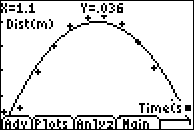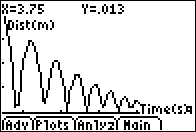# Activities

••• ##### Subject Area

• Math: Calculus: Other Functions

• ##### Author9-12

60 Minutes

• ##### Device
• TI-83 Plus Family
• TI-84 Plus
• TI-84 Plus Silver Edition
• ##### Accessories

CBR™/CBR 2™
TI Connectivity Cable

## Analysis of a Bouncing Ball#### Activity Overview

In this activity, students use the motion detector to collect data for a bouncing ball. They fit an equation to the data with a quadratic regression and use the first and second derivatives to analyze the graphs of velocity and acceleration as a function of time. They discover the connections between the graphs and the motion of the ball.

#### Key Steps

•Students will predict the height from the ground versus time graph for a ball bouncing underneath the motion detector. Then students will use the CBR to collect data from the ball bounce.

•Students will find a quadratic regression equation for the data.